# Parallel coordinates chart with ggally

`ggally` is a `ggplot2` extension. It allows to build parallel coordinates charts thanks to the `ggparcoord()` function. Check several reproducible examples in this post.

# Most basic

This is the most basic parallel coordinates chart you can build with `R`, the `ggally` packages and its `ggparcoord()` function.

The input dataset must be a data frame with several numeric variables, each being used as a vertical axis on the chart. Columns number of these variables are specified in the `columns` argument of the function.

Note: here, a categoric variable is used to color lines, as specified in the `groupColumn` variable.``````# Libraries
library(GGally)

# Data set is provided by R natively
data <- iris

# Plot
ggparcoord(data,
columns = 1:4, groupColumn = 5
) ``````

# Custom color, theme, general appearance

This is pretty much the same chart as te previous one, except for the following customizations:

• color palette is improved thanks to the `viridis` package
• title is added with `title`, and customized in `theme`
• dots are added with `showPoints`
• a bit of transparency is applied to lines with `alphaLines`
• `theme_ipsum()` is used for the general appearance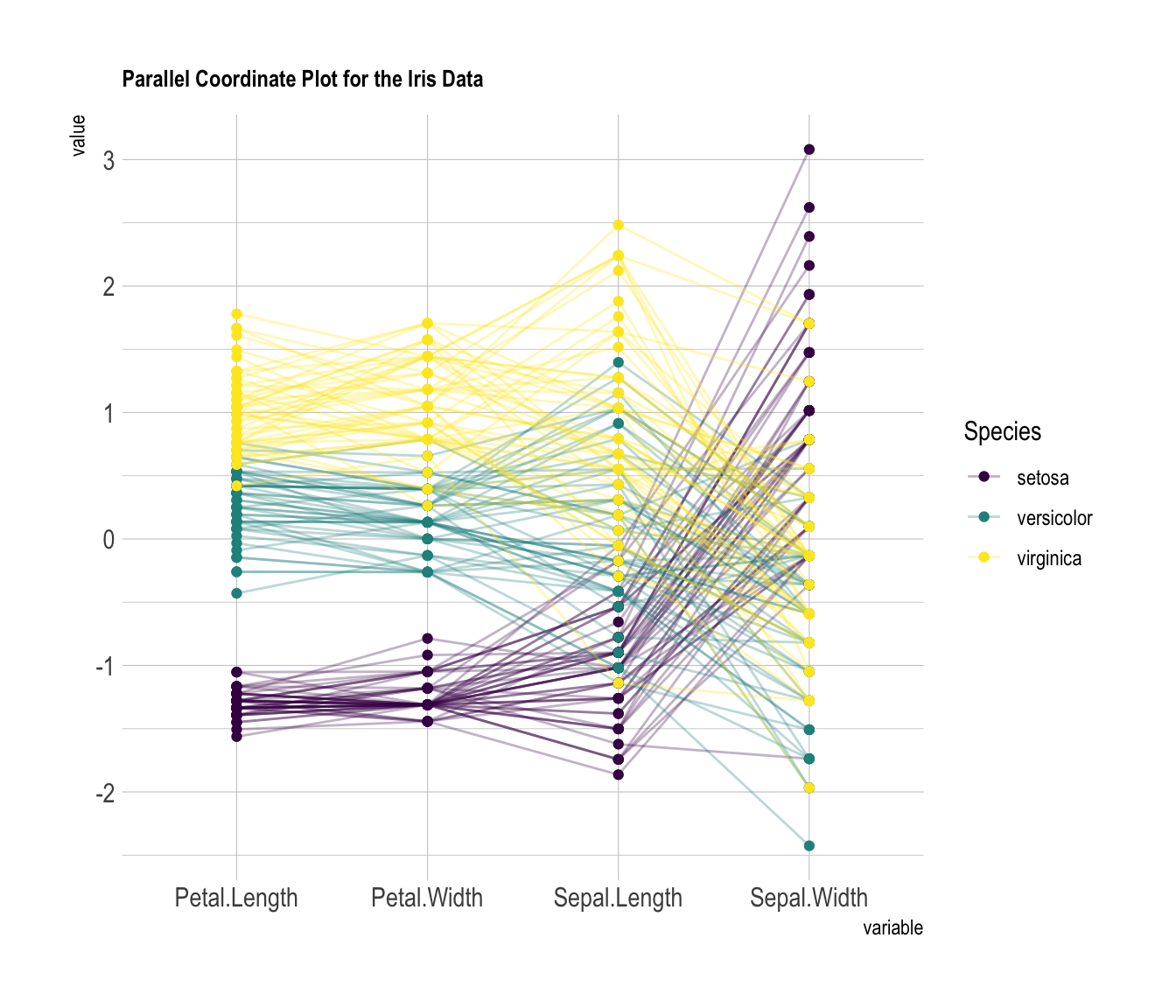``````# Libraries
library(hrbrthemes)
library(GGally)
library(viridis)

# Data set is provided by R natively
data <- iris

# Plot
ggparcoord(data,
columns = 1:4, groupColumn = 5, order = "anyClass",
showPoints = TRUE,
title = "Parallel Coordinate Plot for the Iris Data",
alphaLines = 0.3
) +
scale_color_viridis(discrete=TRUE) +
theme_ipsum()+
theme(
plot.title = element_text(size=10)
)``````

# Scaling

Scaling transforms the raw data to a new scale that is common with other variables. It is a crucial step to compare variables that do not have the same unit, but can also help otherwise as shown in the example below.

The `ggally` package offers a `scale` argument. Four possible options are applied on the same dataset below:

• `globalminmax` → No scaling
• `uniminmax` → Standardize to Min = 0 and Max = 1
• `std` → Normalize univariately (substract mean & divide by sd)
• `center` → Standardize and center variables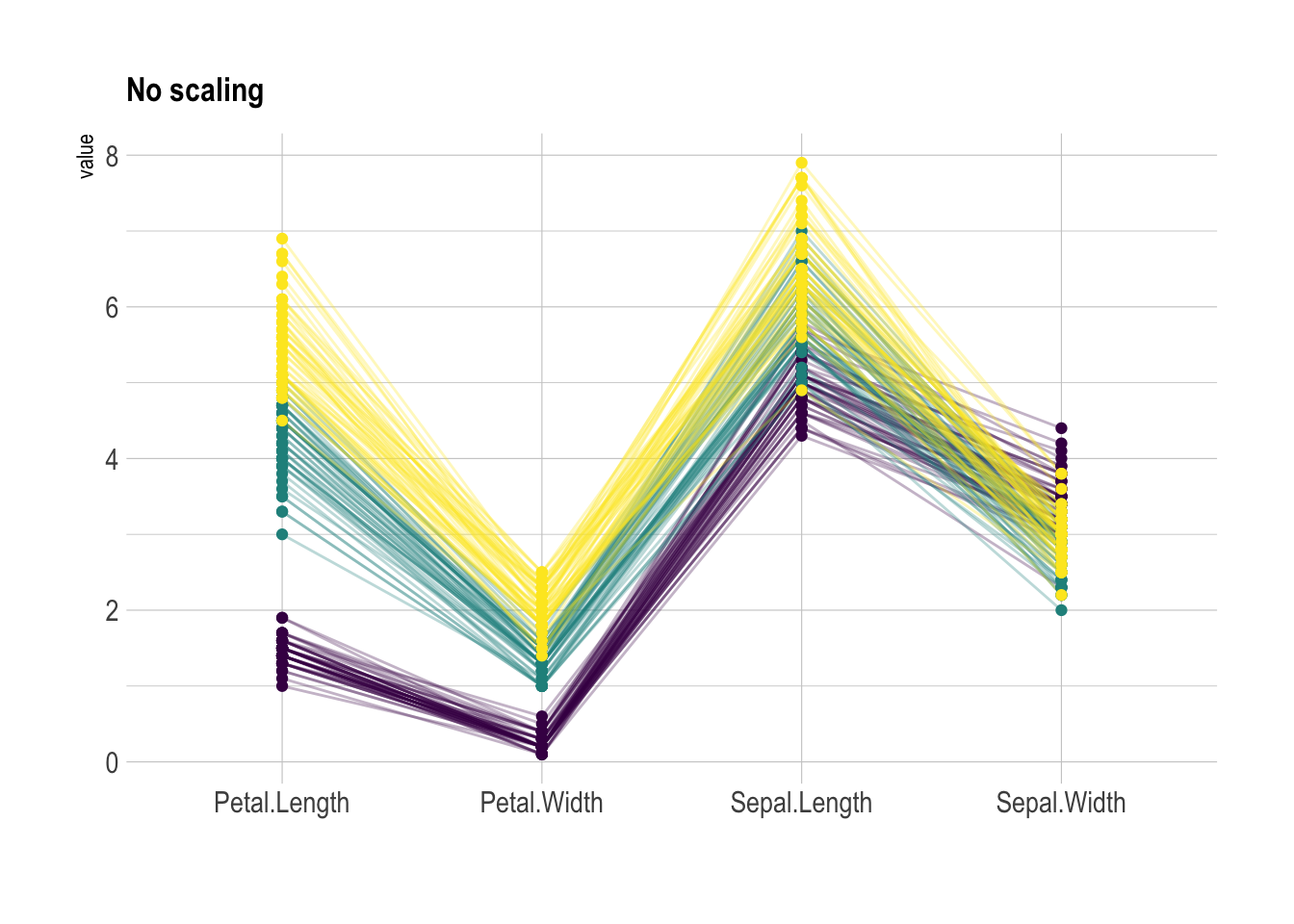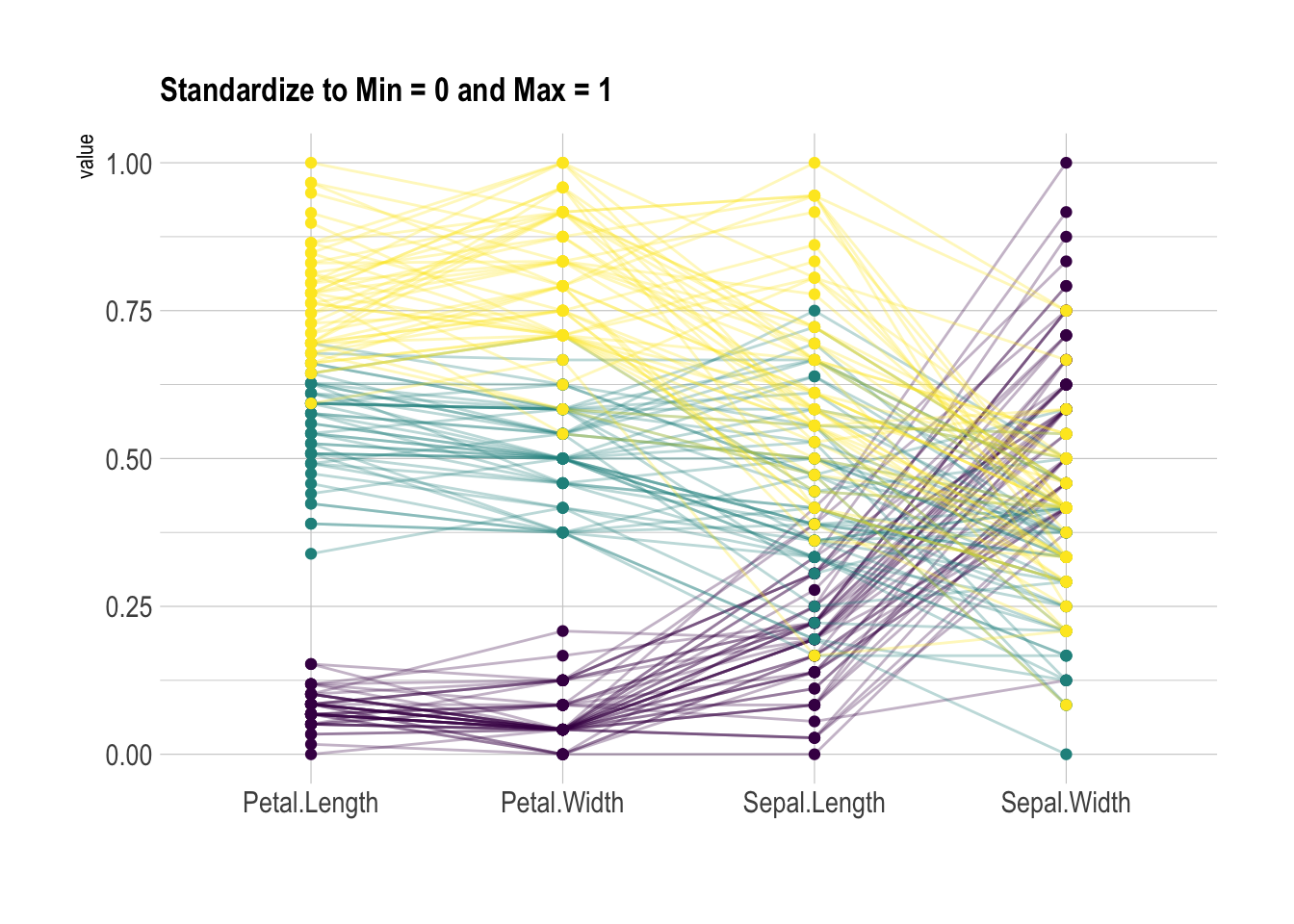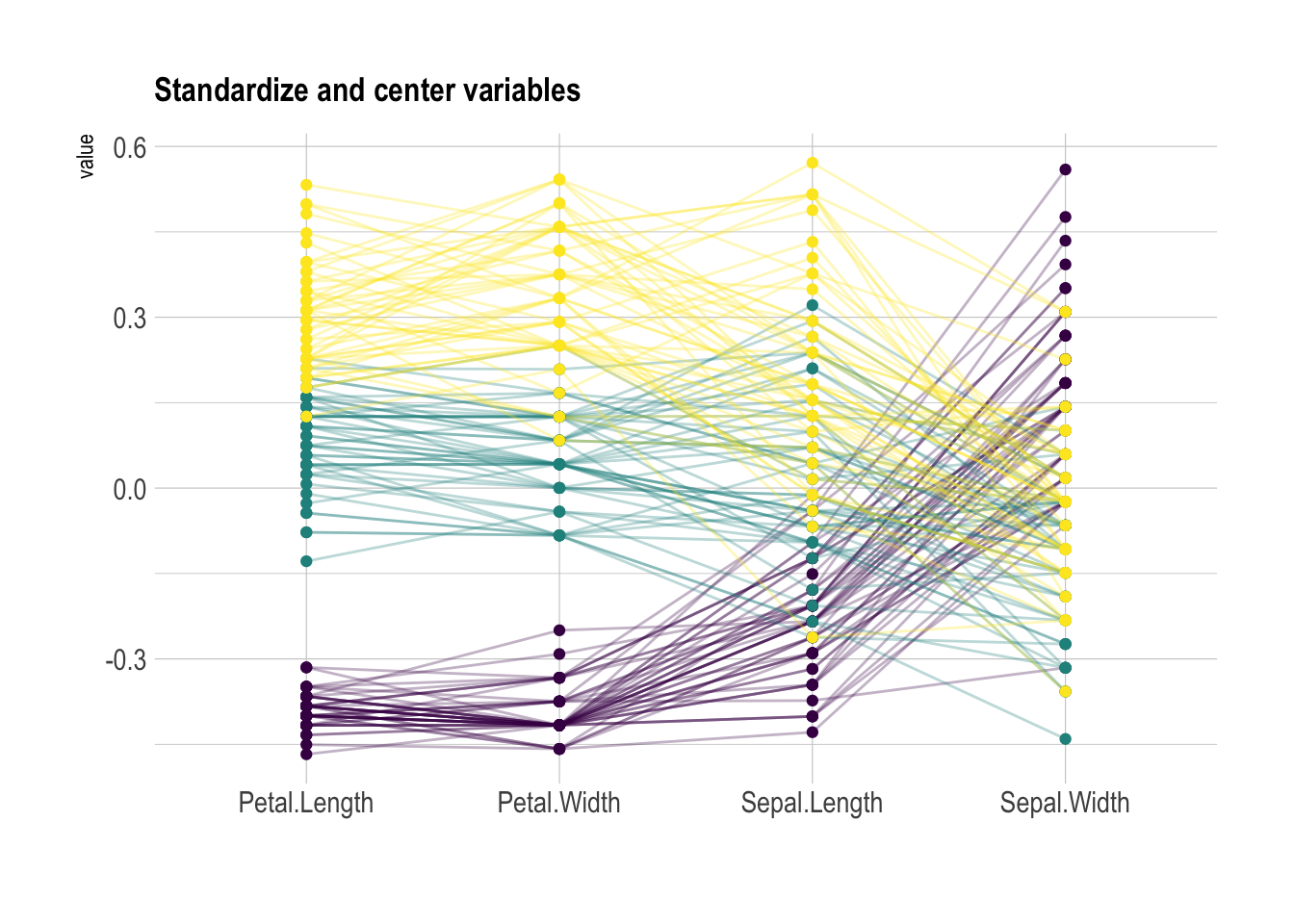``````ggparcoord(data,
columns = 1:4, groupColumn = 5, order = "anyClass",
scale="globalminmax",
showPoints = TRUE,
title = "No scaling",
alphaLines = 0.3
) +
scale_color_viridis(discrete=TRUE) +
theme_ipsum()+
theme(
legend.position="none",
plot.title = element_text(size=13)
) +
xlab("")``````
``````ggparcoord(data,
columns = 1:4, groupColumn = 5, order = "anyClass",
scale="uniminmax",
showPoints = TRUE,
title = "Standardize to Min = 0 and Max = 1",
alphaLines = 0.3
) +
scale_color_viridis(discrete=TRUE) +
theme_ipsum()+
theme(
legend.position="none",
plot.title = element_text(size=13)
) +
xlab("")``````
``````ggparcoord(data,
columns = 1:4, groupColumn = 5, order = "anyClass",
scale="std",
showPoints = TRUE,
title = "Normalize univariately (substract mean & divide by sd)",
alphaLines = 0.3
) +
scale_color_viridis(discrete=TRUE) +
theme_ipsum()+
theme(
legend.position="none",
plot.title = element_text(size=13)
) +
xlab("")``````
``````ggparcoord(data,
columns = 1:4, groupColumn = 5, order = "anyClass",
scale="center",
showPoints = TRUE,
title = "Standardize and center variables",
alphaLines = 0.3
) +
scale_color_viridis(discrete=TRUE) +
theme_ipsum()+
theme(
legend.position="none",
plot.title = element_text(size=13)
) +
xlab("")``````

# Highlight a group

Data visualization aims to highlight a story in the data. If you are interested in a specific group, you can highlight it as follow: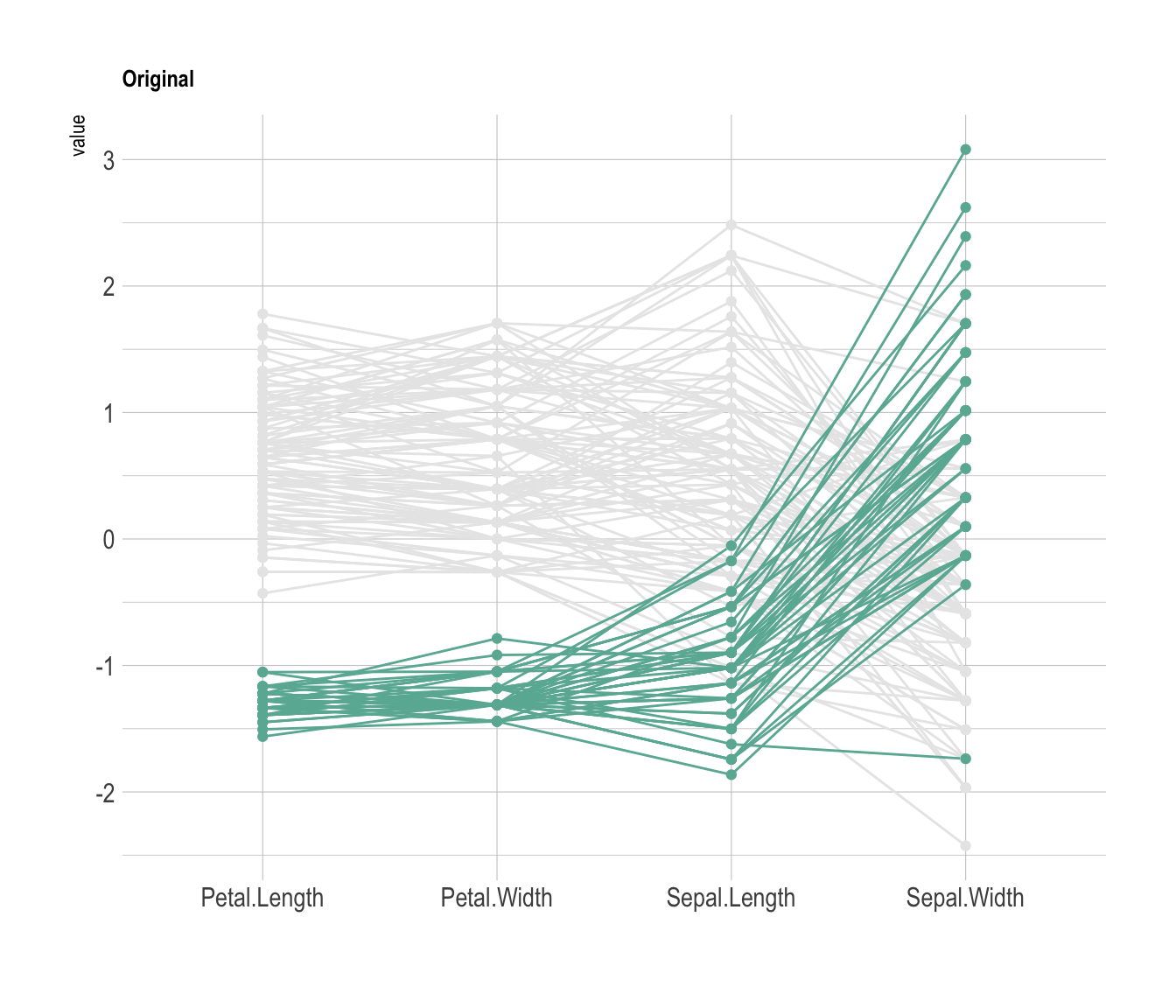``````# Libraries
library(GGally)
library(dplyr)

# Data set is provided by R natively
data <- iris

# Plot
data %>%
arrange(desc(Species)) %>%
ggparcoord(
columns = 1:4, groupColumn = 5, order = "anyClass",
showPoints = TRUE,
title = "Original",
alphaLines = 1
) +
scale_color_manual(values=c( "#69b3a2", "#E8E8E8", "#E8E8E8") ) +
theme_ipsum()+
theme(
legend.position="Default",
plot.title = element_text(size=10)
) +
xlab("")``````

Related chart types

## Contact

This document is a work by Yan Holtz. Any feedback is highly encouraged. You can fill an issue on Github, drop me a message on Twitter, or send an email pasting yan.holtz.data with gmail.com.